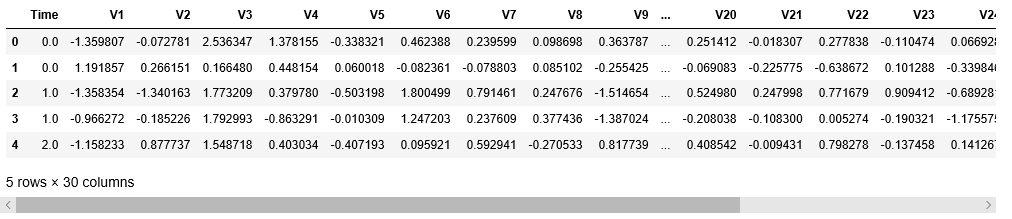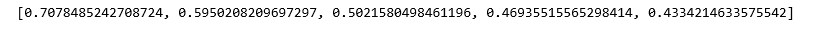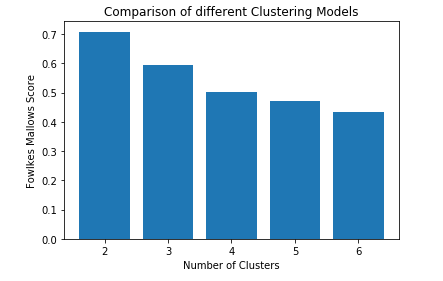# ML | Fowlkes-Mallows Score

The Fowlkes-Mallows Score is an evaluation metric to evaluate the similarity among clusterings obtained after applying different clustering algorithms. Although technically it is used to quantify the similarity between two clusterings, it is typically used to evaluate the clustering performance of a clustering algorithm by assuming the second clustering to be the ground-truth ie the observed data and assuming it to be the perfect clustering.

Let there be N number of data points in the data and k number of clusters in clusterings A1 and A2. Then the matrix M is built such thatwheredetermines the number of data points that lie in the ith cluster in clustering A1 and the jth cluster in clustering A2.

The Fowlkes-Mallows index for the parameter k is given bywhereThe following terms are defined in the context of the above-defined symbolic conventions:-

1. True Positive(TP): The number of pair of data points which are in the same cluster in A1 and in A2.
2. False Positive(FP): The number of pair of data points which are in the same cluster in A1 but not in A2.
3. False Negative(FN): The number of pair of data points which are not in the same cluster in A1 but are in the same cluster in A2.
4. True Negative(TN): The number of pair of data points which are not in the same cluster in neither A1 nor A2.

ObviouslyThus the Fowlkes-Mallows Index can also be expressed as:-Rewritting the above expressionThus the Fowlkes-Mallows Index is the geometric mean of the precision and the recall.

Properties:

• Assumption-Less: This evaluation metric does not assume any property about the cluster structure thus proving to be significantly advantageous than traditional evaluation methods.
• Ground-Truth Rules: One disadvantage to this evaluation metric is that it requires the knowledge of the ground-truth rules(Class Labels) to evaluate a clustering algorithm.

The below steps will demonstrate how to evaluate the Fowlkes-Mallows Index for a clustering algorithm by using Sklearn. The dataset for the below steps is the Credit Card Fraud Detection dataset which can be downloaded from Kaggle.

Step 1: Importing the required libraries

 import pandas as pd  import matplotlib.pyplot as plt  from sklearn.cluster import KMeans  from sklearn.metrics import fowlkes_mallows

 #Changing the working location to the location of the file  cd C:\Users\Dev\Desktop\Kaggle\Credit Card Fraud     #Loading the data  df = pd.read_csv('creditcard.csv')     #Separating the dependent and independent variables  y = df['Class']  X = df.drop('Class',axis=1)     X.head()Step 3: Building different Clustering and evaluating individual performances

The following step lines of code involve Building different K-Means Clustering models each having different values for the parameter n_clusters and then evaluating each individual performance using the Fowlkes-Mallows Score.

 #List of Fowlkes-Mallows Scores for different models  fms_scores = []     #List of different number of clusters  N_Clusters = [2,3,4,5,6]

a) n_clusters = 2

 #Building the clustering model  kmeans2 = KMeans(n_clusters=2)     #Training the clustering model  kmeans2.fit(X)     #Storing the predicted Clustering labels  labels2 = kmeans2.predict(X)     #Evaluating the performance  fms_scores.append(fms(y,labels2))

b) n_clusters = 3

 #Building the clustering model  kmeans3 = KMeans(n_clusters=3)     #Training the clustering model  kmeans3.fit(X)     #Storing the predicted Clustering labels  labels3 = kmeans3.predict(X)     #Evaluating the performance  fms_scores.append(fms(y,labels3))

c) n_clusters = 4

 #Building the clustering model  kmeans4 = KMeans(n_clusters=4)     #Training the clustering model  kmeans4.fit(X)     #Storing the predicted Clustering labels  labels4 = kmeans4.predict(X)     #Evaluating the performance  fms_scores.append(fms(y,labels4))

d) n_clusters = 5

 #Building the clustering model  kmeans5 = KMeans(n_clusters=5)     #Training the clustering model  kmeans5.fit(X)     #Storing the predicted Clustering labels  labels5 = kmeans5.predict(X)     #Evaluating the performance  fms_scores.append(fms(y,labels5))

e) n_clusters = 6

 #Building the clustering model  kmeans6 = KMeans(n_clusters=6)     #Training the clustering model  kmeans6.fit(X)     #Storing the predicted Clustering labels  labels6 = kmeans6.predict(X)     #Evaluating the performance  fms_scores.append(fms(y,labels6))

 print(fms_scores)Step 4: Visualizing and Comparing the results

 #Plotting a Bar Graph to compare the models  plt.bar(N_Clusters,fms_scores)  plt.xlabel('Number of Clusters')  plt.ylabel('Fowlkes Mallows Score')  plt.title('Comparison of different Clustering Models')  plt.show()Thus, quite obviously, the clustering with the number of clusters = 2 is the most similar to the observed data because the data has only two class labels.

My Personal Notes arrow_drop_upCheck out this Author's contributed articles.

If you like GeeksforGeeks and would like to contribute, you can also write an article using contribute.geeksforgeeks.org or mail your article to contribute@geeksforgeeks.org. See your article appearing on the GeeksforGeeks main page and help other Geeks.

Please Improve this article if you find anything incorrect by clicking on the "Improve Article" button below.

Improved By : shubham_singh

Article Tags :
Practice Tags :

Be the First to upvote.

Please write to us at contribute@geeksforgeeks.org to report any issue with the above content.